# Analysis for the Shared-Bike Program in City of Toronto

The Bike Share Toronto System has been put into action since 2017, and we have collected historical ridership data over last four years.

Now, we are analyzing the 4 years of historic data to help inform the program moving forward. Because, we plan to expand the program by moving into new GTA neighborhoods and densifying the existing downtown infrastructure.

There are two datasets of the project, the first one is the Ridership Data which contains 9 fields such as Trip ID, Trip Duration, Bike Station, User Type, and the second dataset is the Weather Data that contains 28 fields such as temperature, Relative Humidity, Wind Speed, visibility. Based on these data, we will have a comprehensive analysis and give some suggestions for future plan. And these are three main part of the analysis. The first part is Data Wrangling and Cleaning where we will Merge this data into a common DataFrame and cleaning it. The Second part is Exploratory Data Analysis where we will explore the dataset to extract insights to help answers some of the City of Toronto’s questions. The third one is modelling where we will develop a simple model for predicting hourly demand.

Then we would like to share the result which we have obtained from the data analysis about the share-bike program in city of Toronto.

Firstly, let us to see the factors that can influence the usage of the share bikes. Based on the result of analysis, we can know that The total number of Annual riders is great than that of Casual riders. There is an increasing trend of usage from 2017 to 2019 and the trend is the same for both Casual, Annual Member and Totoal riders. The number of Annual riders of 2020 is a little less than 2019 which may be resulted from the missing data of November and December 2020.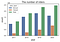The Number of Different Types of Riders from 2017–2020 in the Program

As shown in the picture, there is a different usage behavior between Casual and Annual Member riders. The median duration of Casual trips is great than that of Annual trips. There are more outliers of Annual trips which means that more Annual riders would like to have a longer duration trips.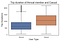Trip Duration Differences about the Annual Member and Casual Mebember of the Program

Due to the fact that it is free to use bikes, and as shown in the figure, the number of riders in Wednesday is the most that may be resulted from the FREE RIDE WEDNESDAYS.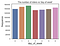The Number of Riders VS The Days in Each Week

We know that the weather has a significant influence on the way people use the bike share system, and more people are decide to have a ride in rain.

Furthermore, to analysis which weather features are most influential, we analyzed the Temperature, Dew point temperature, Relative humidity, the direction from which the wind blows, Wind speed, Visibility, atmospheric pressure, the index to indicate how hot or humid, an index to indicate how cold the weather feels respectively.

In term of temperature, the number of riders increases with the temperature between negative to 20 Celsius degree.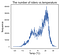The Number of Riders VS. Temperature I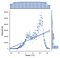The Number of Riders VS. Temperature II

And the number of riders increases with the dew point temperature between negative to 20 Celsius degree.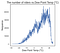The Number of Riders VS. Dew Point Temperature I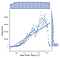The Number of Riders VS. Dew Point Temperature II

In terms of Relative humidity，we know that the number of riders increases with the relative humidity less than 70% and then decrease.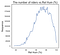The Number of Riders VS. Relative Humidity I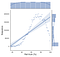The Number of Riders VS. Relative Humidity II

In terms of Wind Direction, as shown in the figure, there is the most riders when the wind direction is around 7.5 degree.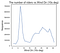The Number of Riders VS. Wind Direction I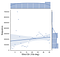The Number of Riders VS. Wind Direction II

In terms of Wind Speed, the number of riders increased with the wind speed when the wind speed ranges from 0 to 15 km/h, and then decreased when the wind speed is large than 15 km/h.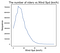The Number of Riders VS. Wind Speed I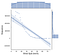The Number of Riders VS. Wind Speed II

And as shown in the figure, the number of riders dramatically increased with the visibility when the visibility is large than 15 km.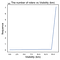The Number of Riders VS. Visibility I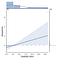The Number of Riders VS. Visibility II

In addition, there are more riders when the atmospheric pressure ranges from 100 KPa to 101KPa.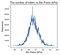The Number of Riders VS. Atmospheric Pressure I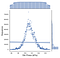The Number of Riders VS. Atmospheric Pressure I

We have also analyzed the Hmdx that is An index to indicate how hot or humid the weather feels to the average person. As shown in the figure, the number of riders increased with the hmdx when the hmdx ranges from 25 to 28, and then decrease with the speed when the wind speed is large than 28.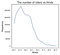The Number of Riders VS. Humid Index I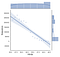The Number of Riders VS. Humid Index II

We also analyzed the Wind Chill, as shown in the figure, there are more riders when the wind chill ranges from -10 to -5.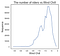The Number of Riders VS. Wind Chill I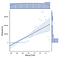The Number of Riders VS. Wind Chill II

Based on the above analysis, we know that the Visibility is the most influential weather feature.

To analyze when people will use the bike share system, we analyzed the usage vary across the year, the week, and the day. As shown in the figure, there is an increase trend of the number of riders from 2017 to 2020.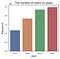The Number of Riders VS. Different Years

And people prefer to have rides from 19th week to 43rd week over one year.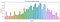The Number of Riders VS. Weeks

In addition, there are more riders who prefer to have rides between 6th and 10th over one month. And the number of riders keep stable from 13th to 30th.

Then, we would make further illustration about the demands for bike, including the general quantity demands for riders, the demands in workdays and holidays. Meanwhile, we would present you about the usage differences for the bike in different area or time.

It is clear in the graph that the number of riders presents increasing trend from 2016–2020 in general. Which means more bikes should be demanded in the future.

After having a specific analysis about the data for recent year, it shows something interesting. In December 2016, there were around 7500 riders only. However, after experiencing some fluctuations, in the Christmas of 2017, the among of riders reached the lowest values, which were 1000 riders at that time. Interestingly, since then, it kept constant increasing even it fluctuated frequently. In June of 2018, it increased to 13 times of the number in 2018 Christmas, which was around 13000 riders. After that, in the August of 2019, the amount of riders reached around 20000 and around 10 months later, the values of riders successfully exceeded to 20000. But what we should notice is that during this 10 months, the values fluctuated greatly and the increasing rate generally slowed down. So, it is necessary for company to invest more funding and put more bikes to the city of Toronto to satisfy the demands of riders. But don’t overinvesting in increasing the bike quantity because the market is getting saturated to some extents. The gradual slowing down increasing rate recently would be the most persuative evidence.

These are five neighborhoods that have seen the largest number of rides depart from bike stations located within their boundaries.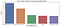The Number of Riders of Top 5 Start Neighborhoods

These are five neighborhoods that have seen the largest number of rides end at bike stations located within their boundaries.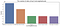The Number of Riders of Top 5 End Neighborhoods

Based on the above conclusion, Waterfront Communities has seen the the largest number of rides depart from and end at bike stations located within its boundaries.

Next, we would like to change our perspective to see the bike usage differences for a workday and a holiday. Firstly, we picked out the Holidays (including Normal Weekends; Christmas Break: 23rd December to January 3rd; Family day; Good Friday; Victoria’s Day; Canada Day; Thanks Giving Day and the Labor’s Day)out because some of them are not fixed, especially for some regional or public holidays. So we picked out these holidays individually from the data set and defined them as holidays. Then, what was left would be the workdays.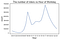The Number of Riders VS. Hour of Workday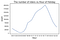The Number of Riders VS. Hour of Holiday

Through the comparison, it is clear that the number of riders in city of Toronto in workdays far more than that in holidays, which is more than four times of that in holidays. More significantly, they presented two completely different distribution shapes.

Now let us to see the bike usage difference for a workday first. It is apparent that it presents a bimodal distribution. And the two peaks shows around 8.00 am and 17.00 pm separately. It perfectly answered the question that what would be the main function for the share-bike, commuting or travelling. In these two rush hours, most of riders would like to use the bike for commuting. So, in workdays, most of riders use the shared-bike for commuting. Meanwhile, it is obvious that the lowest point occurs in midnight. During the period from 10.00 am to 14.00 pm, after experiencing the morning peak, it fluctuates slightly and keep increasing between 14.00 pm and 17.00 pm.

Then, let us focus on the bike usage for a holiday. Unlike the graph for workday, this line graph for holidays only has one summit, which occurs at 17.00 pm in the afternoon. However, unlike the previous one, its lowest point postpones to the early morning, which is around 4.30 am. Then it keeps increasing from 4.30 am until getting the highest points, then it decrease constantly to the 4.30 am in the early morning.

After that, I would analyze the influence of pandemic and city lockdown policy to the usage of bike. We take the date March 13th as the boundary because it is the date when University of Toronto announce the closing of campus officially. Through the graph, it is apparent that the bike usage is still keeping increasing after taking the policy of city-locking down. However, an interesting point should be noticed that the amount of bike used in winter decreased slightly, while in other 3 seasons presented increasing trend in the year of 2020. Then, we would like to do more technical illustration about coding to convincing its validility.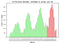The Bike Usage Difference in Toronto Between Lockdown and Normal Period

So, how do we clean the data? We used the weather data, bike share trip data and the station data. One main issue we have with the data is that the trip data contains large number of missing Start Station ID and End Station ID, which constitutes about 12% of the total data. As shown here, the station ids are missing although the station names are there.

So how do we fix that? To recover the data, we use fuzzy match algorithm. We find the closest station name based on bike station data. As shown here, the Queens Park is match with Queen’s Park specified in the station file, Beverly is matched with Beverley with an e. However, fuzzy algorithm does not always work correctly, we may have some errors, like this Shaw St. is matched with John St. To avoid error, we manually define a mapping. After the mapping, the missing stations is reduced from 12% to 1.3%. We just drop the remaining unmatched data.

We also performed other cleaning. We dropped false trips, which correspond to trip with duration smaller than 60s. We removed outliers (2.5% top and 2.5% bottom based on trip duration). From this probability density diagram, we trim the head and tail. We also wrangle “user type” to merge values meaning the same categories. Finally, we convert all time zones to EST.

How do we perform exploratory data analysis? We use visualization to play with the data: 1.Discover important parameters 2.Use statistical model to quantified relationships 3.Train a model. This allows us to discover important parameters. Then, we can use statistical model to quantified relationships and train a model.

For the data modelling, we use a simple multivariable regression model to predict the hourly rides. We have month, day, hour, temperature, humidity, and holiday as the parameters which were shown to have strong correlation with # of trips. We then split the data to 70% training set, 15% validation set and 15% testing set. We use L1 loss function to reduce effect of outlier, and cross-validation for robustness. The model has small least absolute error corresponding to about 20% of the mean, which shows most of the feature can be captured.

After that, we would illustrate about the code of modelling. The Toronto Bike Share hourly rides can be modelling with a simple regression model:

Hourly Rides = a0 + a1*Start Month + a2*Start Day + a3*Start Hour +a4*is_Holiday a5*Temperature, a6*Relative Humidity.

We split the data into 70% training data, 15% validation data and 15% test data.

from sklearn.model_selection import train_test_split

# Split dataset
train, test_val = train_test_split(data_hours, test_size=0.3, random_state=0, shuffle=False)
val, test= train_test_split(test_val, test_size=0.5, random_state=0, shuffle=False)

# Print results
print(‘Train {}%’.format(train.shape / data_hours.shape * 100))
print(‘Val {}%’.format(val.shape / data_hours.shape * 100))
print(‘Test {}%’.format(test.shape / data_hours.shape * 100))

Train 69.99789480015639%
Val 15.001052599921808%
Test 15.001052599921808%

And we train a linear regression model.

from sklearn.linear_model import LinearRegression

# process the data
X_train, y_train = process_data(train)
X_val, y_val = process_data(val)

model = LinearRegression()
model.fit(X_train, y_train)

y_predicted = model.predict(X_val)

print(“RMSE:”, rmse(y_val, y_predicted))
print(“R2 score:”, model.score(X_val, y_val))

RMSE: 283.51492755886085
R2 score: 0.08307002006120612

Opps, we only get a R2 value of 8%! The above model is not good enough. Data science and data modelling is deep!

What happens if we use a different model with L1 loss function?

from sklearn.ensemble import GradientBoostingRegressor

model = GradientBoostingRegressor(loss=’lad’)
model.fit(X_train, y_train)
y_predicted = model.predict(X_val)

print(“RMSE:”, rmse(y_val, y_predicted))
print(“R2 score:”, model.score(X_val, y_val))

RMSE: 258.2076512933231
R2 score: 0.2394591831573264

Just by changing the model, now we have a 24% R2 value!

Let’s try taking casual and annual membership into consideration. We make 2 separate models for each of the user type, and at the end add the two predictions.

# Model for casual members
train, test = train_test_split(data_hours, test_size=0.15, random_state=0, shuffle=False)
print(‘Train {}%’.format(train.shape / data_hours.shape * 100 * (4/5)))
print(‘Validation {}%’.format(train.shape / data_hours.shape * 100/5))
print(‘Test {}%’.format(test.shape / data_hours.shape * 100))

X_train_c, y_train_c = process_data(train, target=’casual_trips’)
X_test_c, y_test_c = process_data(test, target=’casual_trips’)

five_fold = KFold(n_splits=5)
train_index_c, val_index_c = next(five_fold.split(X_train_c))
model_c=GradientBoostingRegressor(loss=’lad’)
model_c.fit(X_train_c.iloc[train_index_c], y_train_c.iloc[train_index_c])
y_predicted_c = model_c.predict(X_train_c.iloc[val_index_c])

print(‘RMSE scores: {}’.format(rmse(y_train_c.iloc[val_index_c], y_predicted_c)))
print(‘R2 scores: {}’.format(model_c.score(X_train_c.iloc[val_index_c], y_train_c[val_index_c])))

# Model for annual members
X_train_a, y_train_a = process_data(train, target=’annual_trips’)
X_test_a, y_test_a = process_data(test, target=’annual_trips’)

five_fold = KFold(n_splits=5)
train_index_a, val_index_a = next(five_fold.split(X_train_a))
model_a=GradientBoostingRegressor(loss=’lad’)
model_a.fit(X_train_a.iloc[train_index_a], y_train_a.iloc[train_index_a])
y_predicted_a = model_a.predict(X_train_a.iloc[val_index_a])

print(‘RMSE scores: {}’.format(rmse(y_train_a.iloc[val_index_a], y_predicted_a)))
print(‘R2 scores: {}’.format(model_a.score(X_train_a.iloc[val_index_a], y_train_a[val_index_a])))

# Combine the two types:
from sklearn.metrics import r2_score

total_val_y = y_train_a.iloc[val_index_a] + y_train_c.iloc[val_index_c]
total_predicted_y = y_predicted_a + y_predicted_c

print(‘RMSE scores: {}’.format(rmse(total_val_y, total_predicted_y)))
print(‘R2 scores: {}’.format(r2_score(total_val_y, total_predicted_y)))

RMSE scores: 118.2376378456563
R2 scores: 0.24447903562429285

The R2 score has only improved by 0.5%. This may be due to the small role casual members play in the total trips. Now let’s compute the test score:

# Model for casual members
y_predicted_c = model_c.predict(X_test_c)
y_predicted_a = model_a.predict(X_test_a)
total_predicted = y_predicted_a + y_predicted_c
total_y_test = y_test_c + y_test_a
print(‘RMSE scores: {}’.format(rmse(total_y_test, total_predicted)))
print(‘R2 scores: {}’.format(r2_score(total_y_test, total_predicted)))

RMSE scores: 368.22469879239424
R2 scores: 0.2805603159958352

In the final test, R2 gets larger than the value obtained during validation. This means we did not overfit the model. By rejecting the oldest data (data used for validation), the model seems to perform well on the most recent data. However, the RMSE gets larger. This reflects the increases in rides in the most recent period (potentially due to covid). The unexpected increase is not captured in the historical data and thus it adds large error to the root-mean-square-error.

Eventually, some suggestion for future share bike program. First, for data collection, better data can improve our model. It would be great to incorporate more casual user types (for single trip, 24 hour pass and 72 hours pass). It would also be good to have user participation program to use GPS data for routes data, as well as price data. Secondly, after understanding the nature of the trips, flexible pricing can be implemented. To have different pricing for weekend vs weekday, rush hours vs non-rush hours, in different geographic locations and perhaps in different weather. This allows consideration of economical elasticity to improve the bike program.

Note: The data used for analyzing is provided by https://bikesharetoronto.com/ Bike Shared Toronto Websites.